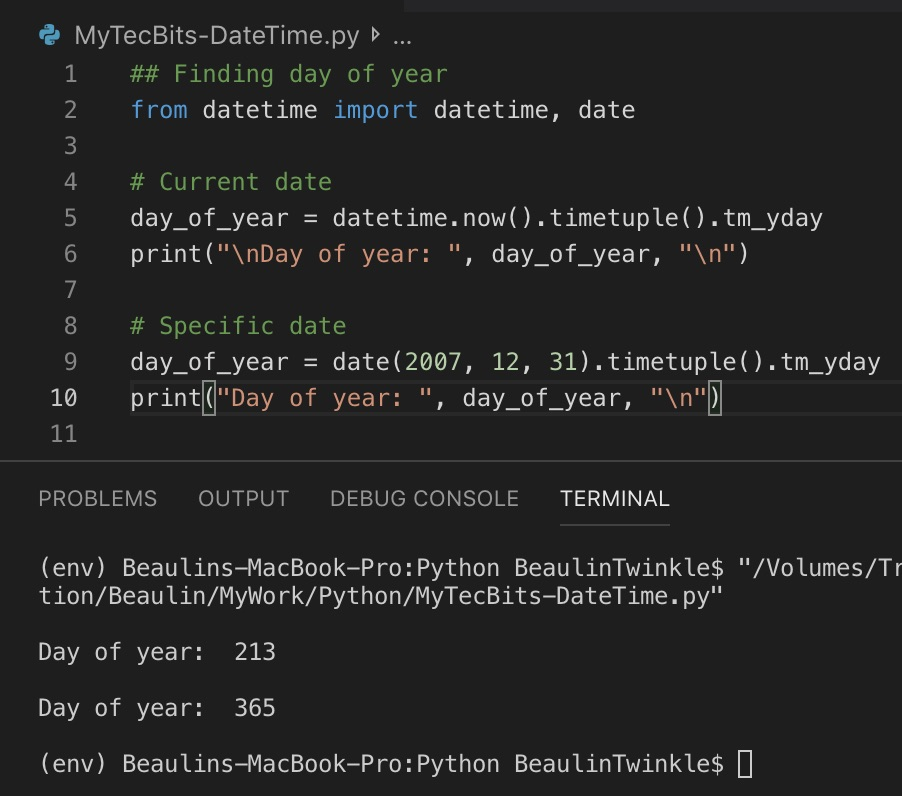# Getting day of year for a given date in Python

There are several ways to get the day of year for a given date in Python programming. Here are few of the methods.

## Method (1) Using timetuple

timetupil() is a method in datetime module which returns a named tuple with several date and time attributes. One among the attribute is tm_yday which is the day of the year. Here is an example using timetupil().

```## Finding day of year
from datetime import datetime, date

# Current date
day_of_year = datetime.now().timetuple().tm_yday
print("\nDay of year: ", day_of_year, "\n")

# Specific date
day_of_year = date(2007, 12, 31).timetuple().tm_yday
print("Day of year: ", day_of_year, "\n")
```

## Method (2) Using toordinal

In this method, we are using the toordinal() method. toordinal() returns the count of days from the first day of Georgian calendar. Using this, we can get the count of days from 01/01/0001 for a specific date and then subtract with the first date of the year of specific date to get the day of year. Here is an example.

```## Finding day of year
from datetime import date

date_val = date(2007, 7, 23)

day_of_year = date_val.toordinal() - date(date_val.year, 1, 1).toordinal() + 1

print("\nDay of year: ", day_of_year, "\n")
```

## Method (3) Using strftime

Another way is to use the strftime(). In strftime() there is a directive %j which returns the day of the year. Here is an example.

```## Finding day of year
from datetime import date

date_val = date(2007, 7, 23)

day_of_year = date_val.strftime('%j')

print("\nDay of year: ", day_of_year, "\n")
```

## Reference

### Related Articles & Tools

This site uses Akismet to reduce spam. Learn how your comment data is processed.